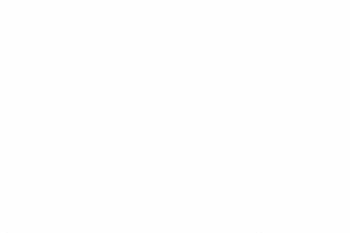# Mathematics Open Book Exam1. Using properties of determinant, show that:2. In any triangle ABC, state and prove tangent law and thence prove that the triangle is either isosceles or right angled if:3. Which center (orthocenter, circumcenter, in center, centroid divides the triangle into 3 small triangles of equal area? Give Reason. Verify your answer analytically. Find the equation of the sides of a triangle having (4,-1) as a vertex and if the lines x-1=0 and x-y-1=0 are the equation of two internal angle bisectors of its angle.

4. Extend your perception about the concept of derivative of a function. Connect it with your daily life. Also extend geometrical interpretation and approach. Find the derivative of:5. find the integration of6. A patient suffering from some known disease has some symptoms similar to covid-19 patients and some of the symptoms occurred 15 days after he attended a cultural program in the city Center. Can we conclude that the patient is suffering from covid-19? Explain using logic.

*****